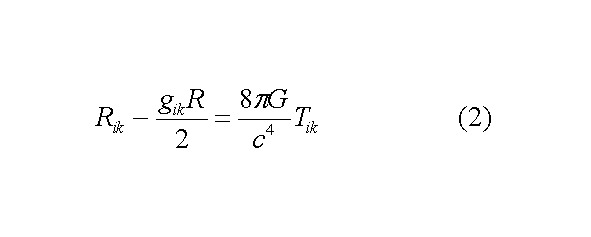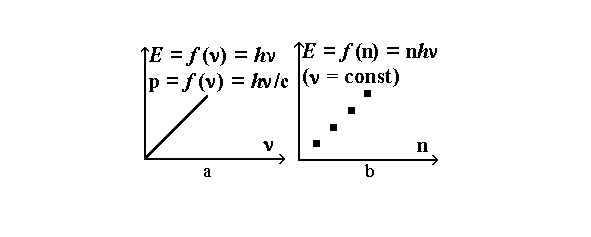электронная
400
печатная A5
499
12+# Бесплатный фрагмент - Quantum theory of gravitation

Объем:
64 стр.
Возрастное ограничение:
12+
ISBN:
978-5-0051-6109-3
электронная
от 400
печатная A5
от 499

Quantization of gravitational field

Abstract:

I derive equations of quantum theory of gravitation containing all 3 fundamental constants — G, c and h.

I introduce and critically analize a new approach to quantization of energy-momentum tensor Tik which fully correlates with experiments.

I derive quantum solution of singularities’ problem in general relativity, maximal density of quantum matter and maximal curvature of spacetime. I show that quantum gravitational collapse is not unlimited compression; for star of any big mass it stops at the stage of maximal density of quantum matter ~1027 kg/m3 with maximal spacetime curvation ~102 m-2 without formation of singularity inside black hole.

The model predicts inevitable big bang after big crunch.

1. Avoid stereotyping

Newton’s theory of gravitation has only one fundamental constant — gravitational constant G. Relativistic theory of gravitation (general relativity) has 2 constants — gravitational constant G and speed of light c. Quantum theory of gravitation by definition must have all 3 fundamental constants — G, c and Planck constant h. Exactly such theory is derived here.

I derive description of gravitational interaction at quantum level, quantum solution of singularities’ problem in general relativity, maximal density of quantum matter and maximal curvature of spacetime. The model predicts inevitable big bang after big crunch.

And, in spite of absence of experimental observations, just using analogy, stereotyping with photon, a graviton hypothesis was put forward. Graviton is postulated as a hypothetical quantum of gravitational field, which is postulated as Bose-condensate of virtual gravitons.

However, during more than a century since derivation of general relativity, all attempts to describe gravitational field as Bose-condensate of virtual gravitons failed and had fundamental difficulties. And all such theories are non-renormalizable and don’t solve the problem [1—21], e.g. a recent review . And it’ particularly noteworthy that the only renormalizable variant  uses fake particles. Therefore the contemporary approach is experimentally inapproppriate. And graviton was not yet found experimentally [22—28].

Therefore we should think in the opposite direction.

And many physicists admit necessity of fundamentally new approaches to derivation of quantum theory of gravitation:

“there are fundamental structural and conceptual mismatches between general relativity and quantum theory. This raises the tantalizing possibility that radical new ideas may be needed to construct a quantum theory of gravity.” 

“The problem of finding and understanding the relationship between quantum theory and gravitation is an extremely difficult one...The difficulties, however, are such that we might well need radical changes in our views or completely new ideas before the problem can be solved.” 

In order to make the greatest scientific discoveries, great scientists of the past always were induced to go against the generally accepted opinion, against dogmas and challenging the crowd stereotypes, e.g.:

* Copernicus, Bruno and Galileo proved heliocentrism while everyone believed in geocentrism. And they were right.

* Columbus sailed West in search of India with the belief that the Earth was spherical, when the whole world considered the Earth flat. And Columbus was right, too!

No matter who you take — Magellan, Newton, Maxwell, Planck, and other geniuses — none of the great scientists was a dogmatist, did not obey the herd instinct. Because dogmatism clings to the old ideas and prevents the creation and even understanding of a new scientific discovery that has already been created. That is why dogmatists do not make discoveries, but even do not understand the pioneers.

Similarly, to realize a scientific discovery, a breakthrough in any field, we will have to do as all geniuses did — move away from the crowd and overcome our herd instinct — after all, we are not a herd of sheep to follow the crowd, but we are thinking people, Homo Sapiens. Therefore, we should be guided by reason, and not by animal herd instinct.

To form such an approach, we need critical thinking and a departure from the usual patterns and stereotypes in thinking:

“A person with an uncritical mind tends to consider the first solution to a problem that comes to mind as final…

Flexibility of mind, which means freedom of thought from preconceived assumptions and template solutions, the ability to find new solutions when the situation and conditions of the problem change.

The flexibility of the mind is expressed not only in freedom from the binding influence of stencil techniques, but also in the ability to diversify attempts to solve, not to repeat those attempts, the wrongness of which has already been discovered. Many people do poorly at solving problems, mainly because they go back to the method that first came to their mind again and again in search of a solution, although each time they are convinced that this method does not lead to anything. Here a kind of “inertia” of thought is revealed: a person does not know how to move his thought from the path it once took. " 

So let’s use critical thinking and let’s move away from the herd instinct, template thinking and stereotyping.

2. The cause of quantization of the electromagnetic field is the presence of the elementary electric charge

In order to create the quantum theory of gravitation, I use a fundamentally new, innovative approach — the search for the elementary mass and the elementary energy-momentum tensor, similar to the elementary electric charge in quantum electrodynamics.

The physical cause of the quantization of electromagnetic field is that there is a minimal, elementary electric charge e, such that all other charges are multiples of it:(1) Electric charge is quantized

In nature there is no an electric charge that is not a multiple of the charge of the electron. All charges are multiples of it. The charge of any elementary particle is a multiple of the charge of an electron. The electric charge does not take either chaotic or infinitesimal values, but only strictly discrete values that are multiples of the electron charge.

Therefore, a proton and an electron, having equal elementary charges (modulus), can exchange one virtual photon, so they can make an elementary act of electromagnetic interaction. An elementary electric charge can emit an elementary electromagnetic field — its quantum is a virtual photon.

Therefore elementary electric charge is included in the equations of quantum electrodynamics.

3. A new approach to quantum gravity

Similarly, to derive the equations of quantum gravity, I propose to try a new approach — to search for and measure the elementary, minimum values of mass, energy, and momentum. And these elementary mass, energy, and momentum can be considered as the source of the assumed graviton.

Only after measuring the elementary values of mass, energy, and momentum, these values can be used in the equations of the quantum theory of gravitation.

I want to draw the reader’s attention to an important fact about quantization of energy and momentum.

In general relativity, the source of the gravitational field is the energy-momentum tensor, a measure of the energy and momentum density in a volume:(2) General relativity equations

Therefore, to find the quantum of the gravitational field — the assumed graviton — we need to find the minimal, elementary values of energy and momentum.

Yes, energy and momentum are quantized:(3)

And usually physicists believe that the fact of quantization of energy and momentum (and hence the energy-momentum tensor in general relativity) is an obvious argument in favor of the graviton and the quantum nature of the gravitational field. But any attempt to create a quantum theory of gravitation faces insurmountable difficulties — all these models are non-renormalizable.

So this is a dead end? What is the problem?

The fact is that the question of quantization of energy and momentum is actually much more complicated.

Planck’s constant, unlike electric charge, is neither an elementary energy nor an elementary momentum.

And the frequency \nu in expression (3) can take a variety of values. Yes, electromagnetic waves are quantized, but a quantum of infrared light has less energy than UV, and a quantum of UV light has less energy than an X-ray quantum.

Therefore, the quantum of electromagnetic radiation (3) is not yet an elementary minimum energy, since in nature there are smaller values of radiation quanta of other wavelengths.

Therefore, the question of the existence of elementary energy depends not only on the Planck constant, but also on the question of the existence of an elementary frequency.

This is the question I want to focus the reader’s attention on. This is the question that must be solved in order to create a quantum theory of gravitation.

Therefore, my approach is the following: just as elementary electric charge is necessary to construct QED, so also elementary minimum values of mass, energy, momentum, and frequency are necessary to construct quantum theory of gravitation.

For a more complete understanding, let’s look at the graphs of the dependence of electric charge, energy, and momentum.The electric charge is quantized. Therefore electromagnetic field is quantized.Energy and momentum are quantized in n, but they are not quantized in frequency \nu. Therefore gravitational field is not quantized.Quantized energy spectrum in n and continuous energy spectrum in frequency \nu. Expression “matter is quantized” means quantization of energy and momentum at n.

4. Is there a minimum elementary mass?

The lightest particles with a rest mass are the electron and positron. Is the mass of an electron the elementary mass — the minimum mass that all other masses are multiples of it? Is here the same situation as in the case of an electric charge?

To answer this question, it is enough to calculate the ratio of the masses of all elementary particles to the mass of the electron. If all these relations are equal to natural numbers, then we can say that the mass of the electron is an elementary mass.

However, after making these simple calculations, we can see that these ratios are not natural numbers, for example, the ratio of the muon and electron masses is ~206.768…, the ratio of the proton and electron masses is ~1836.1527…, and so on.

You can double-check these calculations for all particles with a rest mass. As a result of these simple calculations, it is easy to see that, unlike the electric charge, the masses of elementary particles are not proportional to the mass of the electron.(4)

What conclusions can be drawn from these facts?

Can we say that the mass of an electron is an elementary mass based on these facts? Isn’t it that the opposite conclusion follows from these facts?

This means that we can no longer describe the gravitational interaction between an electron and a proton as an exchange of one virtual graviton. A proton is heavier than an electron and its gravitational field is stronger than that of an electron. And stronger in a non-integer number of times ~1836.1527…

Are we reasoning correctly?

Maybe there is a mass of neutrinos and it is the minimum and elementary mass? Or maybe there is a certain elementary particle — the carrier of the minimum elementary mass? Then it is logical to assume that all other particles with a rest mass must be constructed from neutrinos or from such a particle — carrier of elementary mass. If this were true, then this particle with a mass less than the mass of the electron would appear at particle collisions in colliders. However, experiments rather refute than confirm this line of thought [17—19].

Is it possible to assert the presence of an elementary mass on the basis of these data? No, I think.

5. Is there the elementary mass in the relativity theory?

Even if we found an elementary mass in a non-relativistic theory, in relativity, attempts to quantize mass are complicated by the fact that in it the mass depends on the velocity:

Therefore, in the theory of relativity, the question of the existence of an elementary mass depends on the existence of an elementary velocity.

If the velocity is not quantized, not discrete, if there is no elementary minimum value of the velocity, if the velocity has a continuous spectrum of values, then the mass is also non-quantized, non-discrete, and has a continuous spectrum of values.

6. Is the velocity discrete?

How to solve the issue about quantization, discreteness of the speed? Does the hypothesized discreteness of space-time affect the question of hypothesized discreteness of velocity?

Let us first consider the classic case. The speed is v=s/t, where s is the path in space and t is time.

If time or space is discrete, then logically the question of speed discreteness is solved as follows.

1) if both space and time are not discrete, then speed is not discrete too:

Бесплатный фрагмент закончился.
Купите книгу, чтобы продолжить чтение.
электронная
от 400
печатная A5
от 499